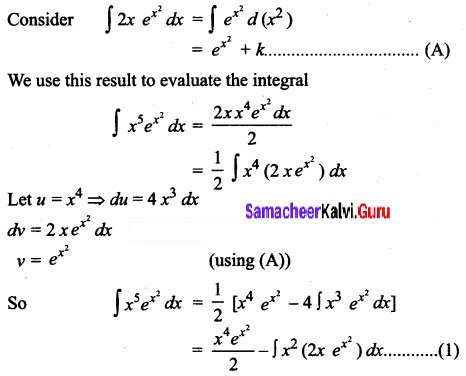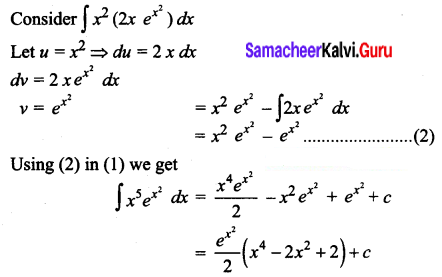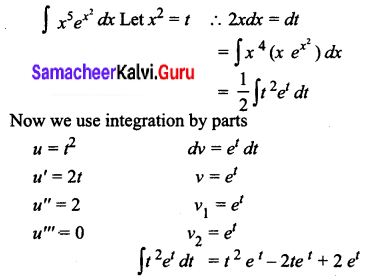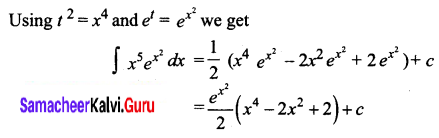# Samacheer Kalvi 12th Business Maths Solutions Chapter 2 Integral Calculus I Ex 2.5

Students can download 12th Business Maths Chapter 2 Integral Calculus I Ex 2.5 Questions and Answers, Samacheer Kalvi 12th Business Maths Book Solutions Guide Pdf helps you to revise the complete Tamilnadu State Board New Syllabus and score more marks in your examinations.

## Tamilnadu Samacheer Kalvi 12th Business Maths Solutions Chapter 2 Integral Calculus I Ex 2.5

Integrate the following with respect to x.

Question 1.
x e-x
Solution: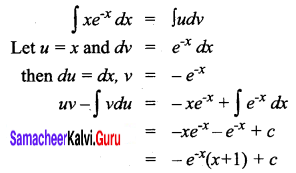Question 2.
x3 e3x
Solution: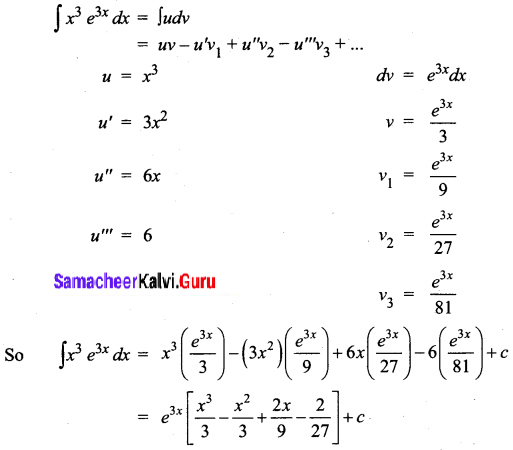Question 3.
log x
Solution: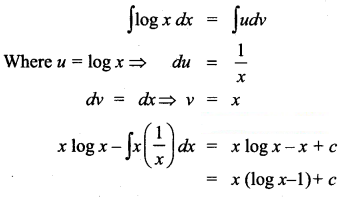Question 4.
x log x
Solution: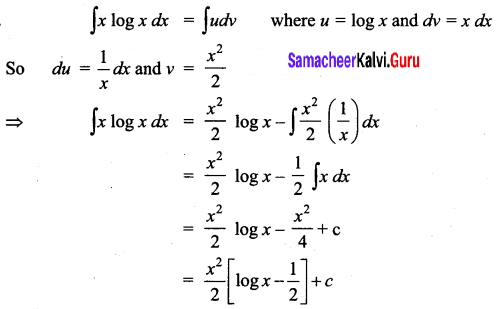Question 5.
xn log x
Solution: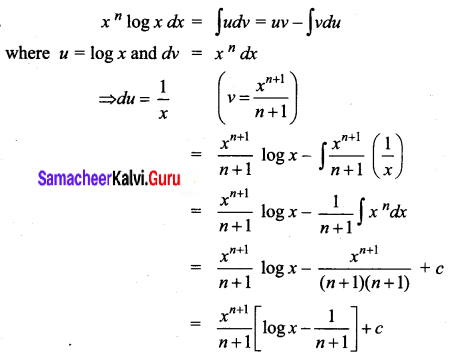Question 6.
$$\boldsymbol{x}^{\boldsymbol{5}} \boldsymbol{e}^{\boldsymbol{x}^{2}}$$
Solution: